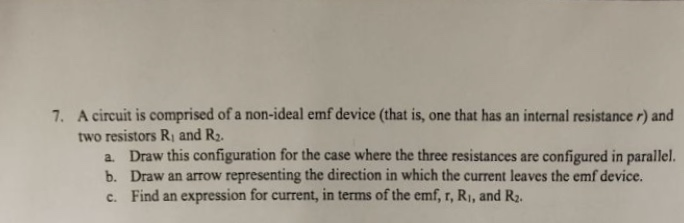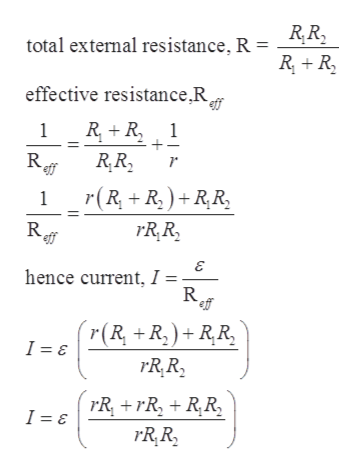# 7. A circuit is comprised of a non-ideal emf device (that is, one that has an internal resistance r) andtwo resistors R, and R2.a. Draw this configuration for the case where the three resistances are configured in parallel.b. Draw an arrow representing the direction in which the current leaves the emf device.c. Find an expression for current, in terms of the emf, r, R1, and R2.

Question
1 viewshelp_outlineImage Transcriptionclose7. A circuit is comprised of a non-ideal emf device (that is, one that has an internal resistance r) and two resistors R, and R2. a. Draw this configuration for the case where the three resistances are configured in parallel. b. Draw an arrow representing the direction in which the current leaves the emf device. c. Find an expression for current, in terms of the emf, r, R1, and R2. fullscreen
check_circle

Step 1

Given:

Two resistors = R1 and R2

Internal resistor = r

Step 2

Circuit can be drawn as:

Step 3

Expression for the curren...help_outlineImage TranscriptioncloseR,R, total external resistance, R = R + R, effective resistance,R eff R, + R, 1 R eff R,R, r(R + R, ) + R,R, R rR,R, hence current, I = R, eff r(R +R,)+ R¸R, I = ɛ rR,R, rR +rR, + R,R, I = E rR,R, fullscreen

### Want to see the full answer?

See Solution

#### Want to see this answer and more?

Solutions are written by subject experts who are available 24/7. Questions are typically answered within 1 hour.*

See Solution
*Response times may vary by subject and question.
Tagged in

### Other